﻿ A Combined Cosmological and Gravitational Redshift Supports Electron-Positron Annihilation as the Most Likely Energy Source of the CMBPublications are Open
Access in this journal
Article Versions
Export Article
• Normal Style
• MLA Style
• APA Style
• Chicago Style
Research Article
Open Access Peer-reviewed

### A Combined Cosmological and Gravitational Redshift Supports Electron-Positron Annihilation as the Most Likely Energy Source of the CMB

Jarl-Thure Eriksson
International Journal of Physics. 2019, 7(1), 16-20. DOI: 10.12691/ijp-7-1-3
Received December 12, 2018; Revised January 22, 2019; Accepted January 29, 2019

### Abstract

A study based on a new formulation of the Friedmann-Lemaître-Robertson-Walker equation has led to an alternative scenario for the evolving universe. Further, a theory which replaces the cosmological constant with the momentum pressure of emerging matter has been developed. The set of equations is called the CBU model (Continuously Breeding Universe). The model offers a tool to investigate the present state of the universe. It is assumed that expansion is caused by the continuous addition of matter, due to, say, electron-positron fluctuations. As a feature of the model the gravitational constant G is inversely proportional to the scale factor a. A gravitational uphill for light coming from distant galaxies causes a redshift far more dominant than the cosmological redshift. However, the influence is small in the nearby space of the Milky Way. The combined redshift provides the time of the CMB maximum. The redshift is zCMB = 1,54·109 for a scale factor of aCMB = 2,55·10-5. According to the CBU theory the time as measured from the initial event, i.e. the birth of the universe, is 389.000 years, surprisingly close to the Standard model value of 380.000 years. The combined redshift zCMB corresponds precisely to the frequency 160,23 GHz and the energy of the two photons emerging from a positron-electron annihilation, 1,63742·10-13 J.

### 1. Introduction

Following known laws of physics and strict mathematical procedures a model of the expansion of the universe has been formulated. It is called the CBU (Continuously Breeding Universe) model, 1, 2. The basic assumptions are: The universe was initiated by a quantum fluctuation of an electrically charged elementary particle and its antiparticle, expansion is due to a continuous generation of particle-antiparticle pairs. The positive energy of matter and radiation is the origin of space, outside the universe there is no space, nothing. The positive energy is balanced by an equal amount of negative potential gravitational energy. The space is curved into itself, the curvature radius being directly proportional to the size of the universe.

The theory of continuous matter creation was proposed by Fred Hoyle in the 1940ies, 3, and independently by Hermann Bondi and Thomas Gold, 4. This was called the steady state theory. The addition of matter was assumed to keep the energy density constant while the universe was undergoing a continuous expansion. In 1974 Paul Dirac suggested that nucleons are created uniformly throughout space, a phenomenon he called additive creation, 5.

If matter and accordingly energy is increasing, there must be a balancing mechanism in the form of negative energy. Alan Guth has, as cited by Lawrence Krauss, 6, proposed the idea, that all energy sums up to zero, negative energy is provided by potential gravitational energy.

Based on the Robertson-Walker metric a comprehensive derivation of the Friedmann acceleration equation was accomplished in ref. 2. The unstable equation required the introduction of a "bang-factor" ß which accounted for the excess pressure responsible for the expansion. Acceleration is extremely high at the beginning, but decreases monotonically to a very modest value of gexp = 1,066·10-11 m/s2 today. It was shown that the cosmological constant in the original Friedmann-Robertson-Walker equation could be replaced by a function of β and r, the radius of the observable universe. A unique finding of ref. 2 was that dark energy, and thereby the cosmological constant, in fact originates from a momentum pressure caused by the continuous addition of new matter.

For a long time there have been speculations about the source of the energy behind the Cosmic Microwave Background (CMB). According to the ruling theory the radiation stems from the surface of the last scattering. The CBU suggests that the energy is due to electron-positron annihilations. The model states that the gravitational constant is inversely proportional to the radius r meaning, that gravitation was much stronger in the past. CMB photons have to climb a steep hillside before they reach us. In the present study we derive the equation for the gravitational redshift. Further we combine it with the cosmological redshift. The redshift will provide us with the information needed to relate the present CMB temperature, TCMB = 2,72648 K, to the Planck black-body spectrum.

### 2. Hypotheses

In addition to the general assumptions presented in the Introduction the CBU theory is based on two important hypotheses. The first one is related to the Large Number Hypothesis (LNH) proposed by Paul A. M. Dirac in 1937, 7. Before him Herman Weyl, 8, and Arthur Eddington, 9, had paid attention to “a coincidence” connecting the universe to the microcosmos. If one squared the ratio of the radius of the universe ru and the estimated radius of the proton rp, one would get a very large number N corresponding to the ratio of the universe mass Mu and the proton mass mp: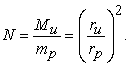(1)

Even if the motive to find a direct relation between the universe and the micro world was somewhat obscure, the fact that the energy of matter would be proportional to the radius squared was considered more than just a coincidence. This leads us to the first hypothesis, let’s call it the Weyl-Dirac hypothesis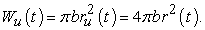(2)

Here Wu is the total amount of positive energy, r = ru/2 is the radius of the observable universe, b is a universal constant to be determined in the next section.

The second hypothesis originates from Mach's principle, after the Austrian physicist Ernst Mach, which influenced Einstein's thinking while working on the theory of General Relativity. According to Mach the collective action of all masses is the cause of inertia. Studying Mach’s principle Dennis Sciama (mentor of Stephen Hawking) concluded in 1953, 10, that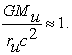(3)

In 1961 Brans and Dicke came to the same conclusion, 11. There is also a simple logical reasoning behind eq. (3). Let us assume that all mass Mu is concentrated to the center of the universe and a small mass m pops out at a distance ru, “the fictitious edge” of the universe. If the energy mc2 is put equal to the potential energy GMum/ru, we end up with eq. (3). Notice that the mass m disappears.

If Mu includes the equivalent mass of radiation, we have Mu = Wu/c2 = 4πbr2/c2, which substituted into eq. (3) leads to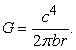(4)

Here the gravitational constant G is inversely proportional to the radius r, a somewhat controversial issue in astrophysics.

### 3. The Size of the Universe

The space of the maiden universe is created by the fluctuation of a positron and an electron, the “straight” distance between these being 2ri. However, due to the curvature of space the interaction distance is πri. Thus the energy equilibrium equation takes the form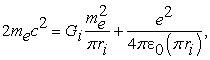(5)

where me and e are the electron mass and charge respectively. Gi is obtained from eq. (3) given that Mu = 2me and ru = 2r = 2ri. We solve ri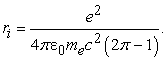(6)

Using known constants of physics we have that ri = 0,533381·10-15 m, a value close to the estimated value of the proton charge radius.

The energy constant of eq. (2) is obtained from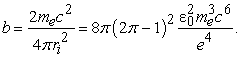(7)

The value is b = 0,458009·1017 J/m2.

From eq. (4) we determine the radius r of the observable universe. The value is r0 = 4,20578·1026 m, slightly smaller than the standard model value of 4,4·1026 m.

### 4. Expansion, New Matter Momentum

Einstein's classical field equation is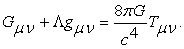(8)

If the energy-momentum tensor Tμν is isotropic and homogeneous an analytic solution can be found. In ref. 2 an expression for the acceleration of universe expansion was derived starting from the Robertson-Walker version of the Friedmann equation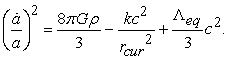(9)

Here a is the scale factor, ρ is the average mass density (radiation included), k is the curvature factor, -1, 0 or 1, and rcur the curvature radius. Λeq is the equivalent cosmological ‘constant’, which here represents the momentum pressure caused by the inflow of new matter.

It appears that the acceleration becomes zero, i.e. the system is in an unstable equilibrium position, a fact also recognized by Einstein. We add a “bang factor” ß to the pressure term in order to account for the cause of expansion, a measure more fruitful than the use of a cosmological constant.

The acceleration equation takes the form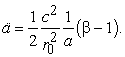(10)

The time derivative of the scale factor a, i.e. expansion velocity, is solved by the integration of eq. (10)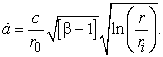(11)

This is the Hubble parameter h. A further integration of eq. (11) leads to a relation between the radius r of the observable universe and the time. The integration, which is presented in ref. 1, comprises an error function solution of the form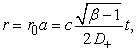(12)

where D+= D+(√ln(r/ri)) is the Dawson integral function. From eq. (11) we have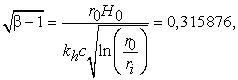(13)

where kh = 3,08567·1022 m/Mpc (H0 = h0kh). In ref. 1 the Hubble parameter was estimated to H0 = 68,24 km/sMpc. We then have ß=1,099778. The age of the present universe appears to be t0 = 4,544·1017 s or 14,40 Gyr, slightly larger than the Standard model age of 13,8 Gyr.

In order for eq. (11) to be consistent with eq (9), and assuming that k = -1 and rcur = r, the equivalent cosmological ‘constant’ must be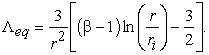(14)

The relation between Λeq and the momentum pressure pP is obtained from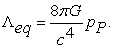(15)

In ref. 2 the pressure was adequately derived from the momentum of incoming new matter, the equivalent expression for ΛeqP differs slightly from eq. (14)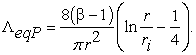(16)

However, it occurs that the difference between Λeq and ΛeqP is only 0,3 % at r = r0 and 2,8 % at r = 10-5r0, the range of r by the time of the CMB, as we shall see in the next section. Thus it is shown that the momentum of new matter quite convincingly explains the dark energy as represented by the cosmological constant Λ in the Standard model. The discrepancy between eq. (14) and eq. (16) is due to the fact that even β is an approximation.

### 5. Redshifts

Our next task is to derive an expression for the redshift caused by gravitation. We write the energy equation for EM radiation climbing a gravitational gradient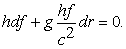(17)

Here f is the frequency, h is Planck’s constant and g the gravitational acceleration along r. The factor hf/c2 may be considered as the virtual mass of the radiation quanta. g is obtained from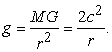(18)

The latter part is obtained by applying eq. (3). Using the relations f = c/λ, df = -cdλ/λ2 and r = r0a we write eq. (17) in the form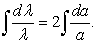(19)

The integration means that λ is proportional to a2. From the following graph

we deduce that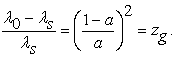(20)

Here zg is the gravitational redshift, λs and λ0 are the wavelengths of the source and the observer respectively.

The the cosmological redshift is obtained from the familiar equation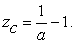(21)

Next we want to show that the combined redshift simply is the sum of zc and zg. Let Δλg= λg0 – λgs= λszg and Δλc= λc0 – λcs= λszc.

Now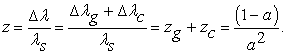(22)

John Huchra at the Harvard-Smithsonian Center for Astrophysics has established the same rule for combining multiple types of redshifts, 12.

An interesting feature of eq. (22) is that the difference between zc and z changes very slowly for scale factors close to 1. The discrepancies of present distance estimates in the vicinity of the Milky Way are still within error limits.

Data on proper distances (d, dpCBU) and lookback times (tΛz, tΛ(zc) and tCBU) are collected in Table 1. Λ refers to the standard ΛCDM model, cf. refs 13 and 14, and CBU to calculated numbers of the present theory.

In Figure 1 the data of Table 1 show the correlation between the ΛCDM and CBU models. There is an astonishingly good agreement between the lookback time graphs. tCBU is based on eq. (12), while tΛz originates from the graph in ref. 13. z was determined from eq. (22) using the appropriate scale factor. dpCMB was calculated according to a unique algorithm developed in ref. 1. Even for the proper distances the similarity between the curves is very good considering the different approaches.

The difference between tΛ(zc) and tΛz is interesting, because it indicates a deviation in the estimates of distant cosmic events. For instance, the most distant observed galaxy GN-z11 has a redshift of 11,09, which would mean that a fully developed galaxy had evolved in about 0,5 Gyr after the Big Bang. This is an intriguing paradox, it is most uncertain that billions of stars would have developed in such a short time. According to CBU the lookback time would be 10,7 Gyr and, considering the age of the universe being 14,4 Gyr, the time for the galaxy to evolve would be a more plausible time of 3,7 Gyr.

• Figure 1. The lookback time and the proper distance as a function of the combined gravitational and cosmological redshift

### 6. The Cosmic Microwave Background

The CMB is commonly considered as the afterglow of the Big Bang. There are, however, divergent opinions about the energy source of this utterly homogenous radiation coming from all directions of space. Charles Lineweaver questions the surface of last scattering, i.e. the recombination of electrons with protons, as the primary source. He suggests that the annihilation of a particle/antiparticle pair is the most logic solution, 15.

Assuming that the CMB radiation is due to electron-positron annihilations the frequency corresponding to the maximum point of the spectrum is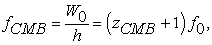(23)

where h is Planck’s constant, W0 = 2mec2 and f0 = 160, 23 GHz as measured today.

From eq. (23) we obtain zCMB = 1,542266·109. The scale factor is according to eq. (22) aCMB = 2,546331·10-5. The redshift differs considerably from the Standard model value of 1089. The time since the initial event as determined from eq. (12) is tCMB = 389.000 yrs. This appears to be very close to the official time of 380.000 yrs.

The brilliance of the Planck black body spectrum is obtained from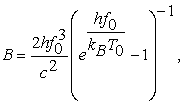(24)

where T0 = 2,72548 K is the present CMB temperature and kB is Boltzmann’s constant. The brilliance of the maximum point is Bmax = 3,83871·10-18 W/(m2srHz) as confirmed by satellite data. The result is dependent on f0, which for a given zCMB is directly connected to the photon energy of an electron-positron annihilation.

### 7. Conclusions

The paper offers a summery of previous studies by the present author. The main purpose is to analyze the influence of a gravitational redshift on time/distance estimations and on the origin of the CMB. Together these studies are an attempt to show that alternative scenarios are available to explain the evolving universe as it appears today. At the bottom we have Einstein’s General Relativity. Sincere proposals by great physicists, such as the radius square law (eq. (2)) related to Dirac’s Large Number Hypothesis and the interpretation of Mach’s Principle, have been compiled to an entity of equations forming the CBU theory.

The relation r = r(t), eq. (12), is almost linear very long back into the past of the universe. An equal feature is also shown in numerous research papers published during the last two decades. Linearity applies at least down to a = 0,15. During a major part of its development the universe does not obey the Friedmann t2/3 law. Further, in ref. 1 it is shown that the light cone diagram, i.e. time to proper length diagram, with great accuracy fits similar graphs, 16, provided that the Hubble constant is H0 = 68,24 km/(sMpc) a value very close to recent estimates.

According to Einstein, curvature is an inherent scalar characteristic of space-time. Apparently the curvature radius changes as the universe expands. Space is not a medium, which expands here and there, it expands uniformly due to the creation of new matter, whereby the curvature radius also increases uniformly. As the interior space of galaxies also expands – at an accelerating pace – we’ll have a Coriolis effect working on all bodies in the galaxy. In ref. 1 it is shown that this effect explains the discrepancies in rotational velocities and thereby excludes the need for dark matter.

In section 4 of this paper the influence of the momentum caused by the inflow of new matter was shortly presented. A rigorous derivation is given in ref. 2. It is quite convincingly shown that the pressure due to the momentum should replace the cosmological constant and that there is no need for dark energy.

The main finding of the present paper is the identification of the gravitational redshift and its effect on the combined redshift. As a result electron-positron annihilation appears to be the obvious energy source of the CMB. It is worth noting that zg was obtained purely on theoretical grounds and that W0 = 2mec2 as the energy source was a consequence. These realities and a matching tCMB are strong evidence in favor of the present study and a changing G. The redshift will have an important impact on time and distance estimations of the universe’s past.

There are still open questions such as the mechanism behind the continuous addition of new matter. But the CBU model does not require any extensions to the already known Laws of Nature nor to the gallery of elementary particles.

Remark: The redshifts presented in ref. 1 do not include gravitational redshift and are therefore incorrect. This does not have any effect on the validity of the CBU theory.

### References

  Eriksson, J.-T., A modified model of the universe shows how acceleration changes galaxy dynamics, International Journal of Physics, vol 6, No. 2, pp. 38-46 (2018): 38-46. In article  Eriksson, J.-T.,The momentum of new matter replaces dark energy and explains the expansion of the universe, International Journal of Physics, vol. 6, no. 5 (2018): 161-165. In article View Article  Hoyle, F., A new model for the expanding universe, Mon. Not. Roy. Ast. Soc., 108, pp. 372-382, 1948 In article View Article  Bondi, H., Gold, T., The steady-state theory of the expanding universe, Mon. Not. Roy. Ast. Soc., 108, pp. 252-270, 1948. In article View Article  Dirac, P., Cosmological models and the Large Number hypothesis, Proc. R. Soc. Lond. A. 338, pp. 439-446, 1974. In article View Article  Krauss, L., Universe from nothing, Simon & Schuster, NY, 2012. In article  Dirac, P. A. M., The cosmological constants, Nature 139, 323, 1937. In article View Article  Weyl, H., Eine neue Erweiterung der Relativitätstheorie, Ann. Phys. 364, 101, 1919. In article View Article  Eddington, A., Preliminary note on the masses of the electron, the proton and the universe, Proc. Cam. Phil. Soc., 27, 1931 from nothing, Simon & Schuster, NY, 2012. In article  Sciama, D. W., On the origin of inertia, Monthly Notices of the Royal Astronomical Society 113, 34-42, 1952. In article View Article  Brans, C., Dicke, R. H., Mach's principle and a relativistic theory of gravitation, Physical Review, 124, 3, pp. 925-935, 1961. In article View Article  Huchra, J., Extragalactic redshifts, NED, NASA/IPAC Extragalactic Database, 2018. In article  Ringermacher, H. I., Mead, L. R., Observation of discrete oscillations in a Model-independent plot of cosmological scale factor vs. lookback time and a scalar field model, arXiv: 1502.06140v1 [astro-ph.CO], 2015. In article  Nemiroff, R., Bonnell, J., Astronomy picture of the day, APOD.NASA, April 8, 2013. In article  Lineweaver, C. H., Inflation and the cosmic microwave background, arXiv: astro-ph/0305179v1, 2003. In article  Davis, T., Lineweaver, C. H ., Expanding confusion: common misconceptions of cosmological horizons and the superluminal expansion of the universe, arXiv: astro-ph/0310808v2, 2003. In articleThis work is licensed under a Creative Commons Attribution 4.0 International License. To view a copy of this license, visit http://creativecommons.org/licenses/by/4.0/